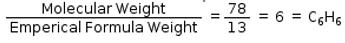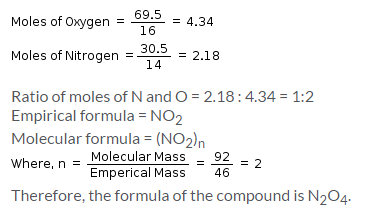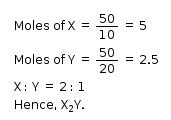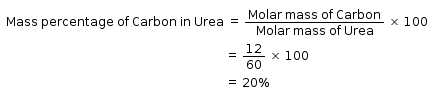Courses

# Test: Formulation & Calculation

## 15 Questions MCQ Test Chemistry for JEE | Test: Formulation & Calculation

Description
This mock test of Test: Formulation & Calculation for JEE helps you for every JEE entrance exam. This contains 15 Multiple Choice Questions for JEE Test: Formulation & Calculation (mcq) to study with solutions a complete question bank. The solved questions answers in this Test: Formulation & Calculation quiz give you a good mix of easy questions and tough questions. JEE students definitely take this Test: Formulation & Calculation exercise for a better result in the exam. You can find other Test: Formulation & Calculation extra questions, long questions & short questions for JEE on EduRev as well by searching above.
QUESTION: 1

### Empirical formula of an organic compound is CH and its molecular mass is 78g/mol. What is its molecular formula?​

Solution:

Empirical formula of mass CH = (12 + 1) = 13
Molecular formula = Empirical formula x nQUESTION: 2

### A compound contains 69.5% oxygen and 30.5% nitrogen and its molecular mass is 92g/mol. The formula of that compound is:​

Solution:QUESTION: 3

### Which formula represents the simplest whole number ratio of various atoms present in a compound?

Solution:

Empirical Formula:

The empirical formula of a substance represents the simplest relative whole number ratio of the atoms of each element contained in the molecule of the substance.

Example: the empirical formula for glucose is CH2O. Thus the molecule of glucose contains atoms of carbon, hydrogen and oxygen in the ratio 1:2:1.

QUESTION: 4

What is the empirical formula of C4H8O2?​

Solution:

Empirical formula represents the simplest whole number ratio of various atoms in a compound hence here n=2 and the empirical formula is C2H4O.

QUESTION: 5

The empirical formula of an organic gas containing carbon and hydrogen is CH2. The mass of 1 litre of this organic gas is exactly equal to that of 1 litre of N2. Therefore, the molecular formula of organic compound is:​

Solution:

Molecular mass of the gas = Molecular mass of N2 = 28 g

Molecular formula mass = n X (Empirical formula mass)

Empirical formula mass of the gas = 12 + 2 = 14 g

Therefore, n = 28/14 = 2

Hence the molecular formula is (CH2)2 or C2H4

QUESTION: 6

Which of the following compounds has the same empirical formula as that of glucose (C6H12O6)?​

Solution:

The empirical formula of a chemical compound is the simplest positive integer ratio of atoms present in a compound.

Molecular formula of glucose is C6H12O6.

Its empirical formula is CH2O.

For CH3COOH, the empirical formula is also CH2O.

QUESTION: 7

The simplest formula of a compound containing 50% of element X (atomic mass = 10g/mol) and 50% of element Y (atomic mass 20g/mol) is:​

Solution:QUESTION: 8

Formula mass is applicable in the case of:

Solution:

The formula unit mass of a substance is a sum of the atomic masses of all atoms in a formula unit of a compound

Both molecular mass and formula mass signify the same thing.

Formula unit mass is the term used for mass of ionic compounds.  Similarly, molecular mass is used for mass of covalent compounds (molecules).

The only reason we use two different terms is because molecules are covalent compounds meaning they have only non-metals. So ionic compounds cannot be a molecule and therefore it would not make sense to find the molecular mass for an ionic compound, so we just call it the "formula mass", when we are talking about ionic compounds.

Molecular mass: For covalent compounds, compounds that contain only non-metals such as SO2, CO2 etc.

Formula mass: For ionic compound, compounds that contain metals and non-metals such as Na2CO3, K2CO3 etc.

QUESTION: 9

What is the empirical formula of C2H2 and C6H6?​

Solution:

Empirical formula represents the simplest whole number ratio of various atoms in a compound hence for both C2H2 and C2H6 the empirical formula is CH.

QUESTION: 10

A compound has an empirical formula of C2HBr. If the molar mass of the compound is 314.7 g/mol, what is the molecular formula of the compound? (Atomic mass of Br = 80g/mol)​

Solution:

Empirical formula mass is (2 X 12) + 1 + 80 = 105g and molar mass of the compound is 314 g/mol.

Hence, n = 3

Therefore, the molecular formula is C6H3Br3.

QUESTION: 11

The empirical formula of an acid CH2O2 is:​

Solution:

Empirical formula of an acid is CH2O2

(Empirical formula) ×n= molecular formular

n = Whole number multiple i.e 1,2,3,4......

if n=1 molecular formula CH2O2

QUESTION: 12

What is the mass percentage of carbon in urea [CO(NH2)2]? (Molar mass of urea is 60g)​

Solution:QUESTION: 13

An oxide of metal M has 40% by mass of oxygen. Metal M has relative atomic mass of 24g/mol. The empirical formula of the oxide is:​

Solution:QUESTION: 14

The empirical formula of a compound is CH2Cl. If the molecular mass of the compound is 99 g, what is the molecular formula of the compound?​

Solution:

Empirical formula mass = 49.5 g.

Molecular mass = 99 g

Therefore n = 2

Therefore molecular formula of compound = (CH2Cl)2

QUESTION: 15

If empirical formula of a compound is C5H4 and the value of n is 2, then molecular formula of the compound is:​

Solution:

Molecular formula of the compound is (C5H4)n
since here n = 2 therefore molecular formula will be C10H8.